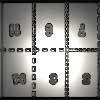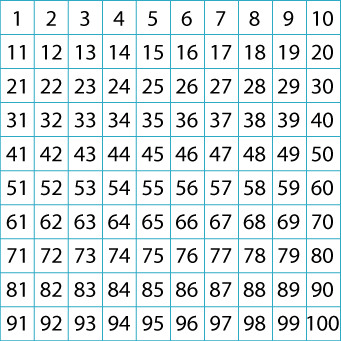#### You may also like### Exploring Wild & Wonderful Number Patterns

EWWNP means Exploring Wild and Wonderful Number Patterns Created by Yourself! Investigate what happens if we create number patterns using some simple rules.### Magazines

Let's suppose that you are going to have a magazine which has 16 pages of A5 size. Can you find some different ways to make these pages? Investigate the pattern for each if you number the pages.### Pebbles

Place four pebbles on the sand in the form of a square. Keep adding as few pebbles as necessary to double the area. How many extra pebbles are added each time?

# Hundred Square

## Hundred Square

A hundred square has been printed on both sides of a piece of paper. One square is directly behind the other.

What is on the back of $100$? $58$? $23$? $19$?

Can you see a pattern?This problem is also available in French: Grille de 100

### Why do this problem?

This problem is an unusual way to explore number patterns in a well-known context. The activity will reinforce the construction of the hundred square, and increase children's familiarity with the sequences contained within it. Using a common resource, such as a hundred square, is a good way for children to begin to use visualisation, which they may find quite difficult at first. The act of visualising in this problem tests children's understanding of how the number square is created.

### Possible approach

You could start by asking the group to close their eyes and try to see a hundred square in their heads. Ask some questions such as "What number comes first and what is below it?"; "What number is below $10$?"; "What number is two places to the right of $34$?". Encourage them to justify their responses before using a real hundred square to check.

You could then pose the question in the problem and encourage the group to work in pairs. Give them time to talk to each other about possible solutions without making a hundred square available at first. [This sheet has two hundred squares on it which may be useful for some children.] Asking pupils to work in pairs on this task will encourage them to begin to argue mathematically. Listening to their explanations and justifications of which numbers are where would be an excellent assessment exercise for you.

When it comes to articulating their method, it is a chance for you to see how well they can put into words what they notice and how they use mathematical understanding and vocabulary to support this. Once the whole group has shared some ways of coming to a solution, pose some further questions for pairs to work on. It is interesting to find out whether some children adopt ways of working on the problem that they learnt from their classmates rather than sticking to their original method. There are many different ways of 'seeing' a solution.

### Key questions

If you turned over the hundred square, where would you write the $1$ of the new hundred square?
So, which number would this $1$ be behind?
Where would the $2$ be? And the $3$?
What number is below $1$ on the hundred square?
Can you imagine the square on the back?
Where will its $1$ be? Where will its $10$ be?
What is on the back of $100$? $58$? $23$? $19$?

### Possible extension

Learners could use a mirror on the hundred square and note all the patterns they can find. What happens if you add each number on the front to the number behind it? Using a different square such as a [$64$] $8$ by $8$ square will produce a different, if related, set of numbers.

### Possible support

Many children will find this task more accessible if they have a real copy of a hundred square to use and annotate as they feel fit. [This sheet has two hundred squares on it.]